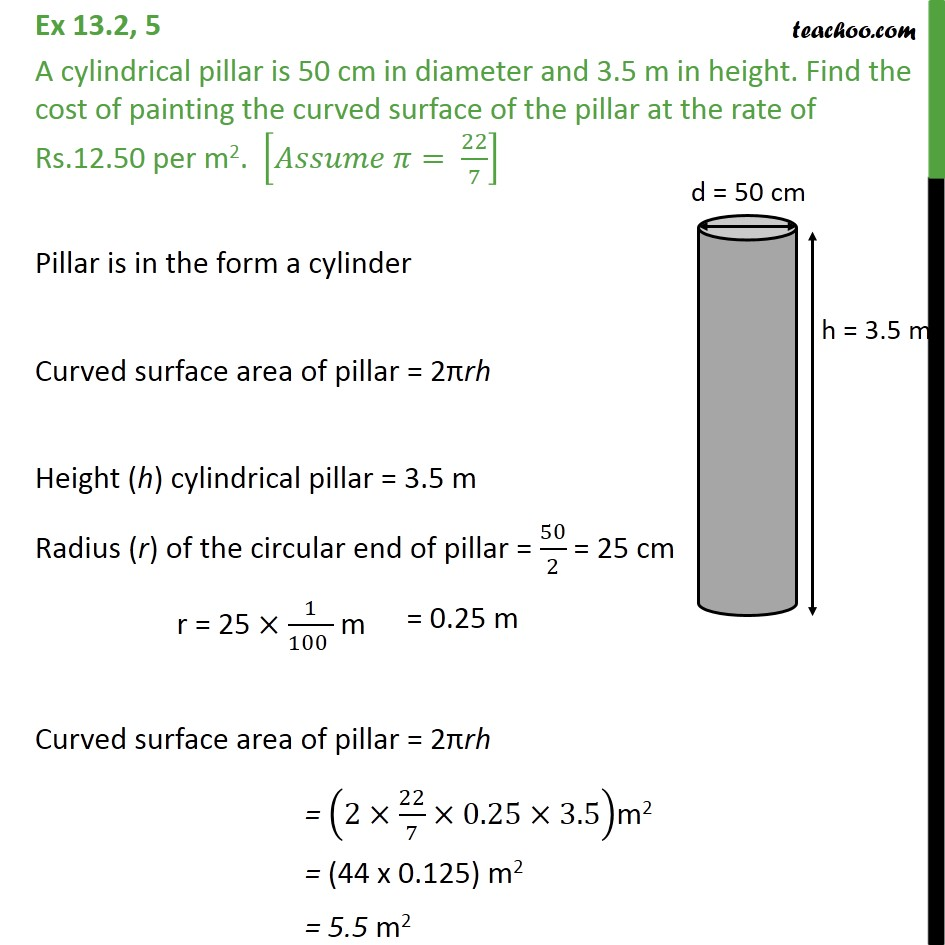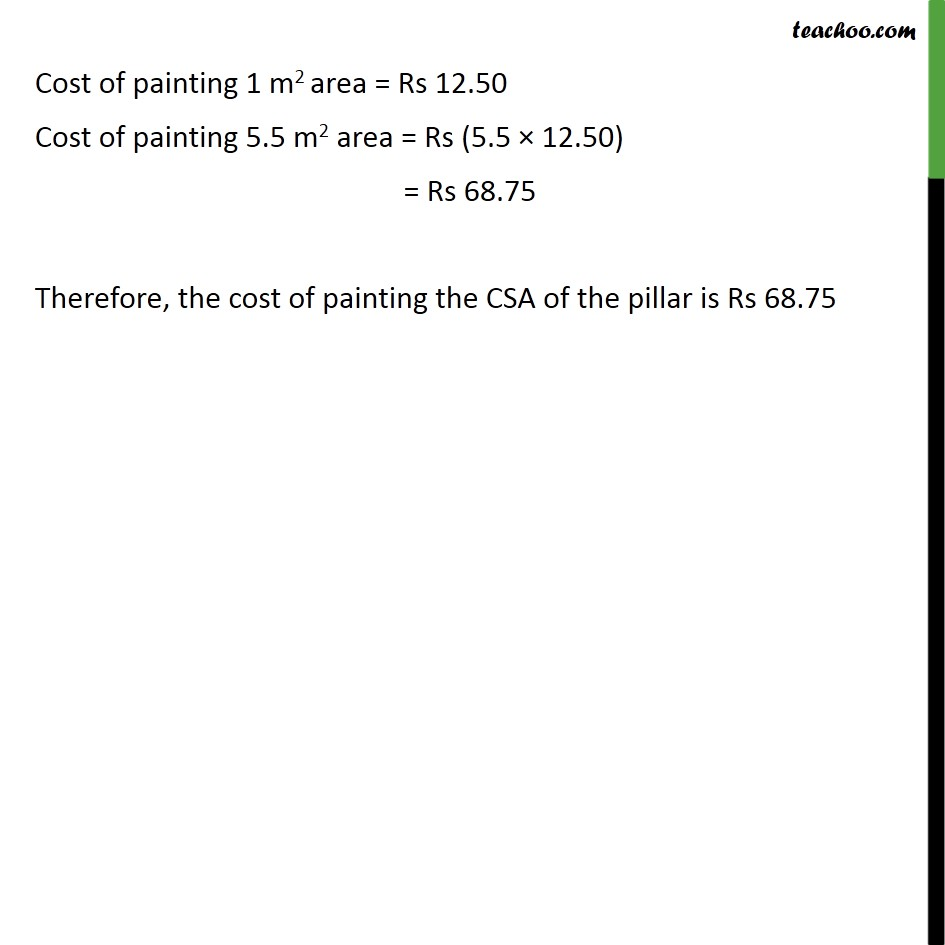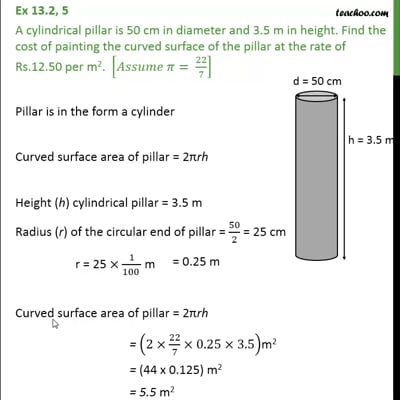Area Of Cylinder

Chapter 13 Class 9 Surface Areas and Volumes
Concept wiseThis video is only available for Teachoo black users

Introducing your new favourite teacher - Teachoo Black, at only ₹83 per month

### Transcript

Ex 13.2, 5 A cylindrical pillar is 50 cm in diameter and 3.5 m in height. Find the cost of painting the curved surface of the pillar at the rate of Rs.12.50 per m2. [ = 22/7] Pillar is in the form a cylinder Curved surface area of pillar = 2 rh Height (h) cylindrical pillar = 3.5 m Radius (r) of the circular end of pillar = 50/2 = 25 cm r = 25 1/(100 ) m Curved surface area of pillar = 2 rh = (2 22/7 0.25 3.5)m2 = (44 x 0.125) m2 = 5.5 m2 Cost of painting 1 m2 area = Rs 12.50 Cost of painting 5.5 m2 area = Rs (5.5 12.50) = Rs 68.75 Therefore, the cost of painting the CSA of the pillar is Rs 68.75